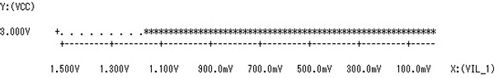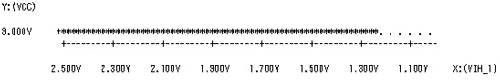﻿ Shmoo Plot Margin Analysis | Reliability Technology Division | Services | OKI Engineering

Location: Home > Services > Tester Program & Device Testing > Measurements and Evaluations > Shmoo Plot Margin Analysis

# Services

## Shmoo Plot Margin Analysis

For example, when analysing CPU malfunction, the cause of defect is often found to be low device input voltage and timing margin, etc. In order to avoid such malfunction, a margin analysis by means of a Shmoo Plot is highly recommended.

A Shmoo Plot is a graph with two axes which plots the magnitude of the dependency on each other as responding to constantly varied correlated parameters such as input timing and supply voltage -- a means of visualization of measurement results.

### Example of Margin Analysis with a Shmoo Plot

Figure 1 and 2 show a Shmoo Plot obtained by measuring the margin against device high (VIH) and low (VIL) level input supply voltages (VCC), while a Shmoo Plot obtained by measuring the margin against supply voltage of input timing (IN1) is shown in figure 3.

From figure 3 we understand that there is a margin of 5ns against an input value of 20ns, demonstrating that a Shmoo Plot, by which we can visualize measurement results, is an effective technique of margin analysis.

Figure 1: SHM • SHEET　Input Voltage Characteristics Shmoo (VIL)
Item Start Stop Step

Inout voltage　X: (VIL_1)

1.5V 0.0V Auto
Supply voltage　Y: (VCC) 3.0V 3.0V -Figure 1 SHM • SHEET　Input Voltage Characteristics Shmoo (VIL)

Figure 2: SHM • SHEET　Input Voltage Characteristics Shmoo (VIH)
Item Start Stop Step

Input voltage　X: (VIH_1)

2.5V 1.0V Auto
Supply voltage Y: (VCC) 3.0V 3.0V -Figure 2 SHM • SHEET　Input Voltage Characteristics Shmoo (VIH)

Figure 3: SHM • SHEET AC Timing Characteristics Shmoo (Input)
Item Start Stop Step

Input timing　X: (IN1)

0.0ns 40ns 0.05ns
Supply voltage Y: (VCC) 3.6V 3.0V -0.1VFigure 3: SHM • SHEET AC Timing Characteristics Shmoo (Input)